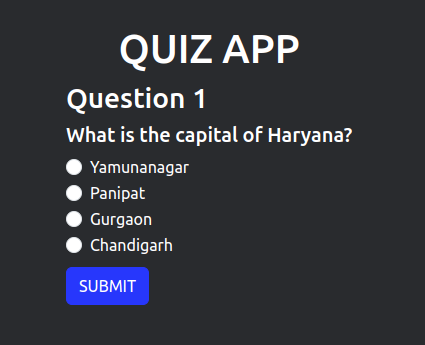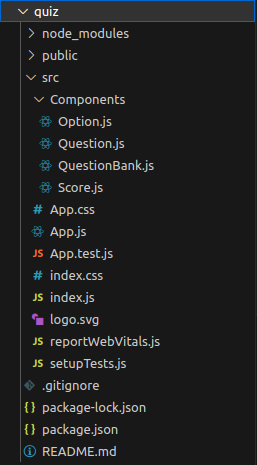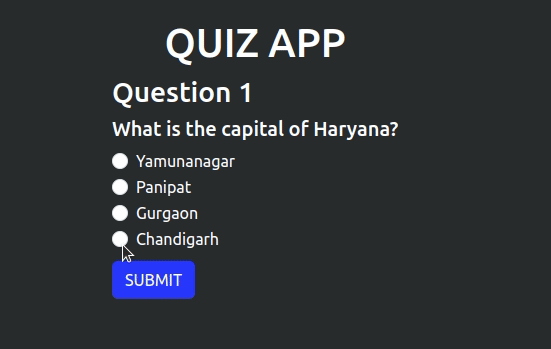Open In App
Related Articles

# Create a Quiz App using ReactJS

In this article, we will create a quiz application to learn the basics of ReactJS. We will be using class components to create the application with custom and bootstrap styling. The application will start with questions at first and then score will be displayed at last. Initially there are only 5 questions but you can keep adding more questions to make the quiz interesting.

Lets have a look at how our final project will look like:Quiz App using ReactJS

Modules required:

Steps to create the project:

Step 1: Create the project file using command.

`npx create-react-app quiz`

Step 2: Navigate to the folder using the command

`cd quiz`

Step 3: Install the required module using the command.

`npm i bootstrap`

Step 4: Create a folder named components in src file and create new files Option.js, Question.js, QuestionBank.js, and Score.js

Project Structure:The dependencies in package.json will look like:

package.json

```"dependencies": {
"@testing-library/jest-dom": "^5.16.5",
"@testing-library/react": "^13.4.0",
"@testing-library/user-event": "^13.5.0",
"bootstrap": "^5.3.0",
"react": "^18.2.0",
"react-dom": "^18.2.0",
"react-scripts": "5.0.1",
"web-vitals": "^2.1.4"
},```

Write the following codes in different files.

Example:

• App.js: This is our main file where all the logic for scoring and questions is written and other components are imported
• Question.js: This component contains the question component to be displayed
• QuestionBank.js: This component contains the raw data of the questions in our file
• Option.js: This component has options corresponding to a particular question
• Score.js: This component is used to display the final soce

## Javascript

 `// App.js` `import React, { Component } from ``"react"``;``import ``"bootstrap/dist/css/bootstrap.min.css"``;``import Question from ``"./Components/Question"``;``import qBank from ``"./Components/QuestionBank"``;``import Score from ``"./Components/Score"``;``import ``"./App.css"``;` `class App extends Component {``    ``constructor(props) {``        ``super``(props);``        ``this``.state = {``            ``questionBank: qBank,``            ``currentQuestion: 0,``            ``selectedOption: ``""``,``            ``score: 0,``            ``quizEnd: ``false``,``        ``};``    ``}` `    ``handleOptionChange = (e) => {``        ``this``.setState({ selectedOption: e.target.value });``    ``};` `    ``handleFormSubmit = (e) => {``        ``e.preventDefault();``        ``this``.checkAnswer();``        ``this``.handleNextQuestion();``    ``};` `    ``checkAnswer = () => {``        ``const { questionBank, currentQuestion, selectedOption, score } = ``this``.state;``        ``if` `(selectedOption === questionBank[currentQuestion].answer) {``            ``this``.setState((prevState) => ({ score: prevState.score + 1 }));``        ``}``    ``};` `    ``handleNextQuestion = () => {``        ``const { questionBank, currentQuestion } = ``this``.state;``        ``if` `(currentQuestion + 1 < questionBank.length) {``            ``this``.setState((prevState) => ({``                ``currentQuestion: prevState.currentQuestion + 1,``                ``selectedOption: ``""``,``            ``}));``        ``} ``else` `{``            ``this``.setState({``                ``quizEnd: ``true``,``            ``});``        ``}``    ``};` `    ``render() {``        ``const { questionBank, currentQuestion, selectedOption, score, quizEnd } =``            ``this``.state;``        ``return` `(``            ``
``                ``

QUIZ APP

``                ``{!quizEnd ? (``                    ````                ``) : (``                    ````                ``)}``            ``
``        ``);``    ``}``}` `export ``default` `App;`

## CSS

 `/* App.css */` `body {``  ``background-color``: ``#292b2e``;``  ``color``: ``#ffffff``;``}` `.app-title {``  ``color``: ``#ffffff``;``  ``margin-top``: ``2``rem;``}`

## Javascript

 `// Question.js` `import React, {Component} from ``"react"``;``import Options from ``"./Option"``;` `class Question extends Component{``    ``render() {``        ``const {question, selectedOption, onOptionChange, onSubmit} = ``this``.props;` `        ``return``(``            ``
``                ``

Question {question.id}

``                ``
{question.question}
``                ``
``                    ````                    ````                ````                ` `            ``
``        ``)``    ``}``}` `export ``default` `Question;`

## Javascript

 `// Option.js` `import React, { Component } from ``'react'``;` `class Options extends Component {``    ``render() {``        ``const { options, selectedOption, onOptionChange } = ``this``.props;` `        ``return` `(``            ``
``                ``{options.map((option, index) => (``                    ``
``                        ````                        ````                    ``
``                ``))}``            ``
``        ``);``    ``}``}` `export ``default` `Options;`

## Javascript

 `// Score.js` `import React, { Component } from ``'react'``;``import ``'../App.css'` `class Score extends Component {``    ``render() {``        ``const { score, onNextQuestion } = ``this``.props;` `        ``return` `(``            ``
``                ``

Results

``                ``

``            ``
``        ``);``    ``}``}` `export ``default` `Score;`

## Javascript

 `// QuestionBank.js` `const qBank = [``    ``{``        ``id: 1,``        ``question: ``"What is the capital of Haryana?"``,``        ``options: [``"Yamunanagar"``, ``"Panipat"``, ``"Gurgaon"``, ``"Chandigarh"``],``        ``answer: ``"Chandigarh"``,``    ``},``    ``{``        ``id: 2,``        ``question: ``"What is the capital of Punjab?"``,``        ``options: [``"Patiala"``, ``"Ludhiana"``, ``"Amritsar"``, ``"Chandigarh"``],``        ``answer: ``"Chandigarh"``,``    ``},``    ``{``        ``id: 3,``        ``question: ``"What is the capital of India?"``,``        ``options: [``"Delhi"``, ``"Mumbai"``, ``"Kolkata"``, ``"Chennai"``],``        ``answer: ``"Delhi"``    ``},``    ``{``        ``id: 4,``        ``question: ``"What is the capital of Uttarakhad?"``,``        ``options: [``"Roorkee"``, ``"Haridwar"``, ``"Dehradun"``, ``"Nanital"``],``        ``answer: ``"Dehradun"``    ``},``    ``{``        ``id: 5,``        ``question: ``"What is capital of Uttar Pradesh?"``,``        ``options: [``"GB Nagar"``, ``"Lucknow"``, ``"Prayagraj"``, ``"Agra"``],``        ``answer: ``"Lucknow"``    ``},``]` `export ``default` `qBank;`

Steps to run the application:

Step 1: Type the following command in your command line

`npm start`

Step 2: Open http://localhost:3000/ URL in the browser. It will display the result.

Output:Quiz App using ReactJS